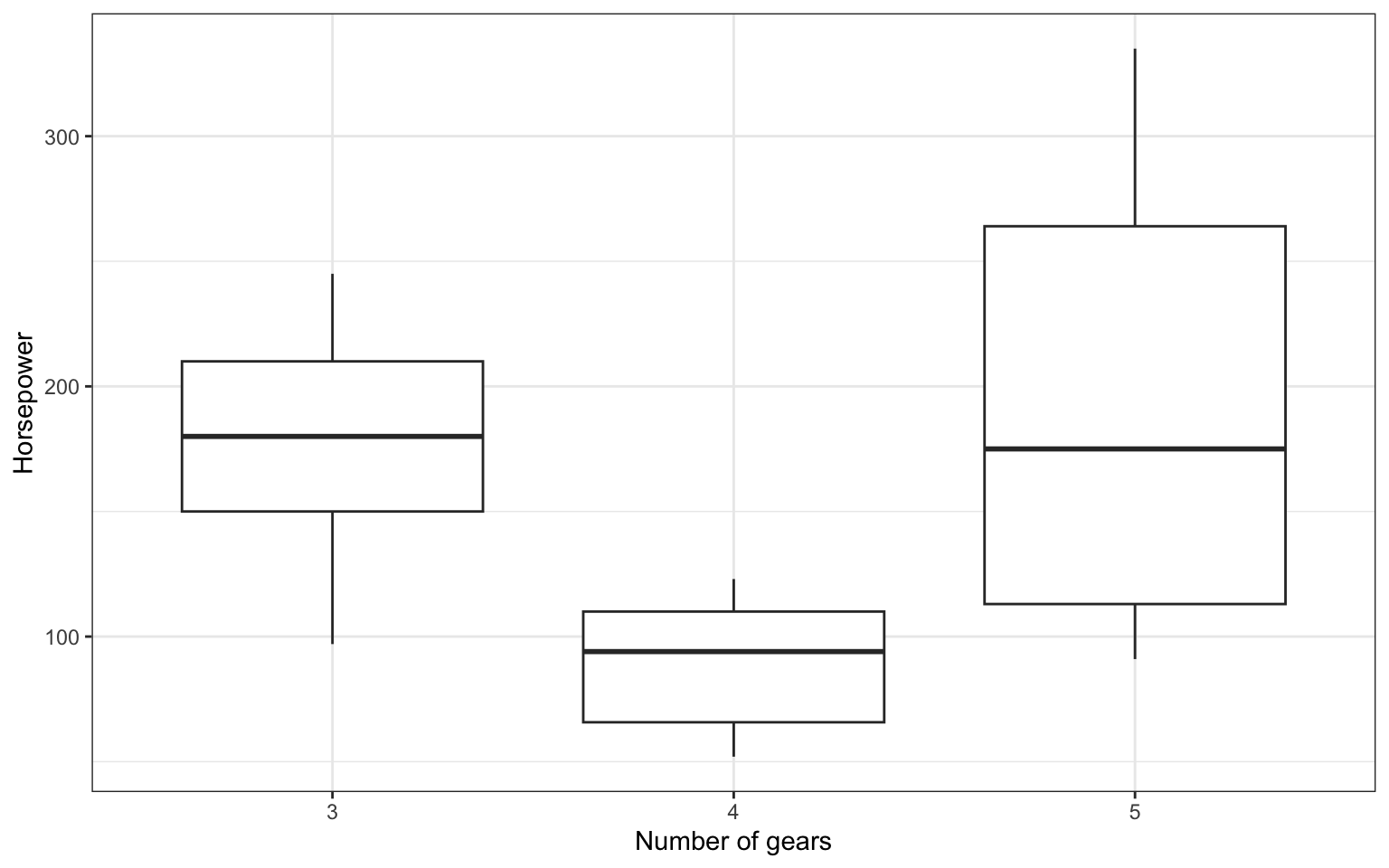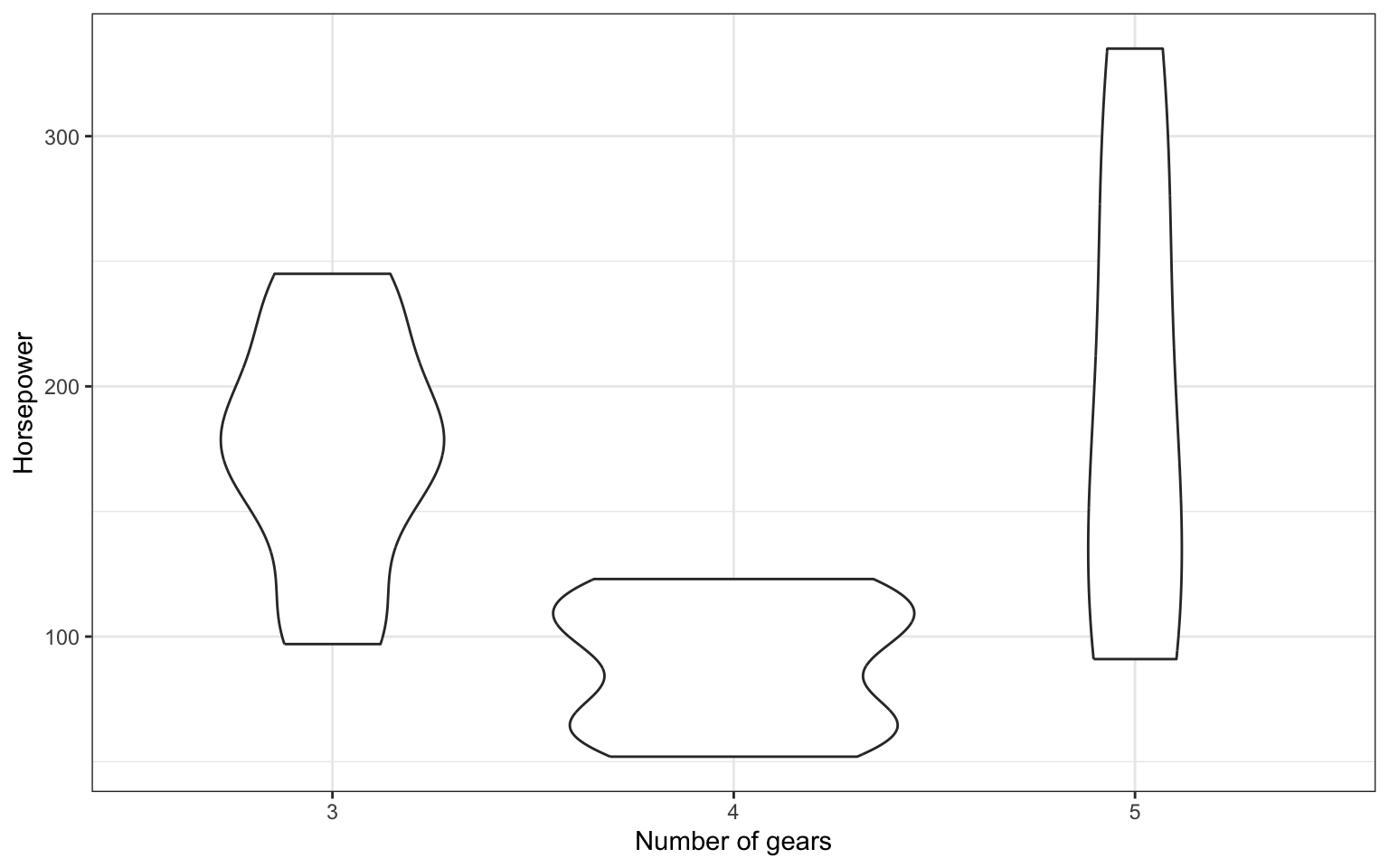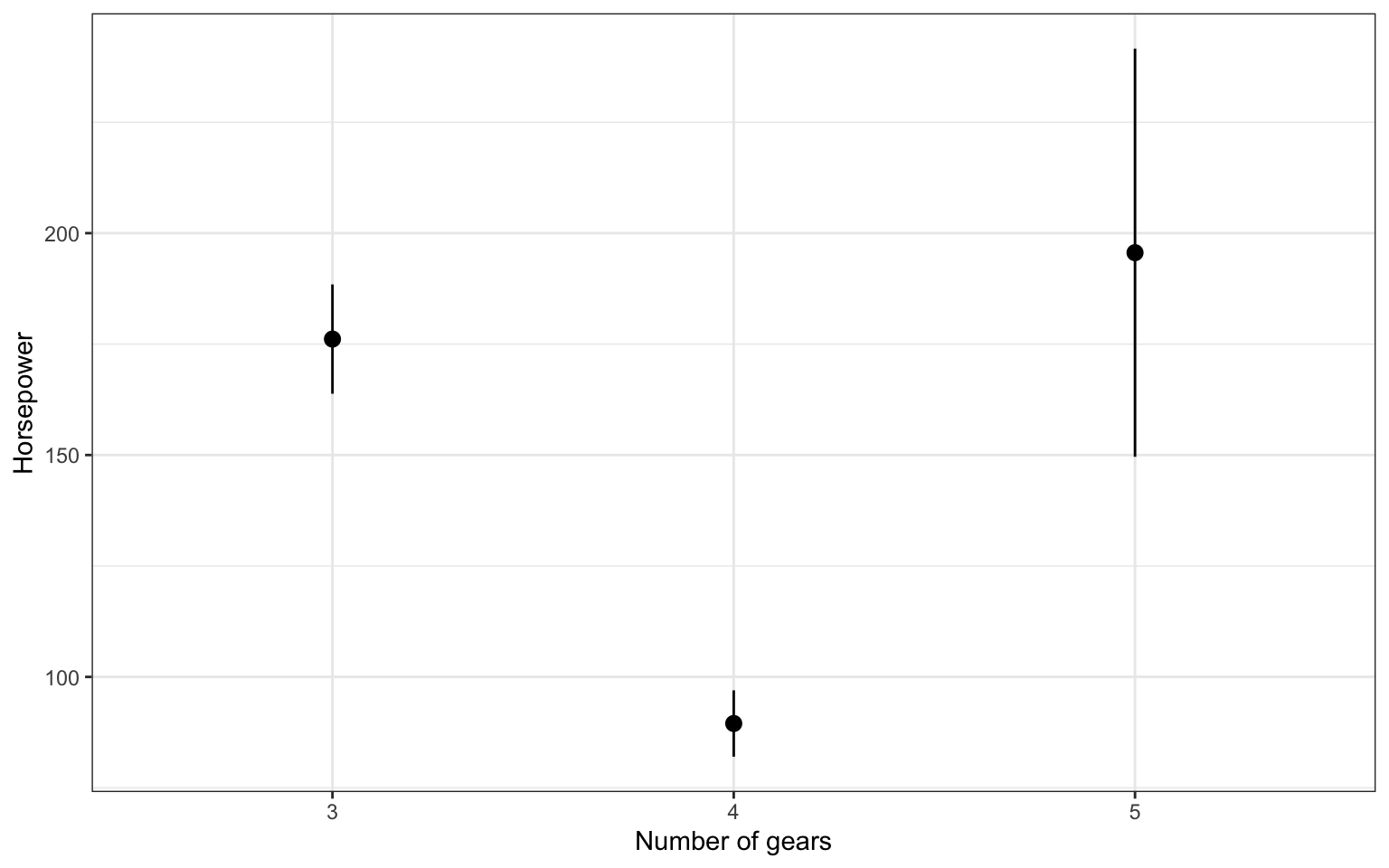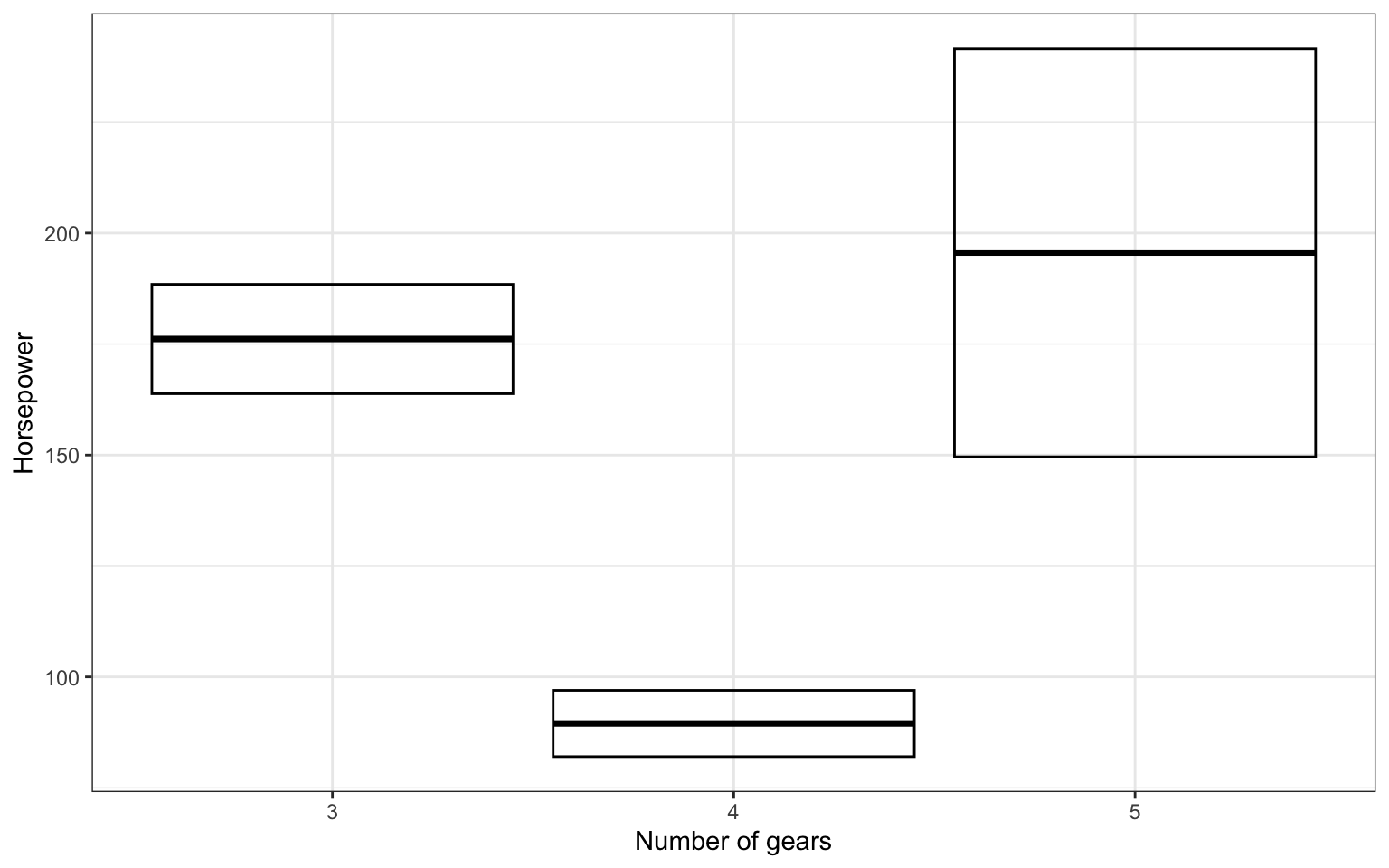# 8 G

## 8.1 general linear model

A mathematical model comparing how one or more independent variables affect a continuous dependent variable

The GLM is a general mathematical framework for expressing relationships among variables that can express or test linear relationships between a numerical dependent variable and any combination of categorical or continuous independent variables.

## 8.2 generalizability

A term referring to the degree to which findings can be readily applied to situations beyond the particular context in which the data were collected.

In the ideal case, we want our findings to be as generalizable as possible. However, when collecting data, we face limitations in the kinds of people, stimuli, and situations we are able to observe. Findings from a study are not generalizable if they do not apply beyond the context in which they are collected. Subjects, stimuli, tasks, and situations are all factors to consider when assessing the generalizability of a study.

## 8.3 geom

The geometric style in which data are displayed, such as boxplot, density, or histogram.

In ggplot2, the geoms control how your data are visualised. You can start with the same base plot...

gg <- ggplot(mtcars, aes(as.factor(gear), hp)) +
labs(x = "Number of gears",
y = "Horsepower")

...and use different geoms to visualise it:

gg + geom_boxplot()Figure 8.1: Visualisation using geom_boxplot

More...
gg + geom_violin()Figure 8.2: Visualisation using geom_violin

gg + stat_summary(geom = "pointrange")Figure 8.3: Visualisation using pointrange

gg + stat_summary(geom = "crossbar")Figure 8.4: Visualisation using crossbar

## 8.4 git

One type of version control software.

Set up git and github with RStudio.

## 8.5 github

A cloud-based service for storing and sharing your version controlled files.

Set up git and github with RStudio.

## 8.6 glm

A mathematical model comparing how one or more independent variables affect a continuous dependent variable

## 8.7 global environment

The interactive workspace where your script runs

When you create a variable, it is usually stored in the global environment. You can view this in the environment tab of the top right pane of RStudio.

If you create a variable inside of a function, it is usually not accessible outside of that function and you won't see it in the global environment tab.

You don't need to know much about environments to use R until you start developing packages; they are covered in Advanced R.